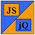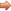# JavaScript & jQuery Tutorials

JS HomeJS IntermediateMore Maths Functions

## More Maths Functions

This lesson follows on from JavaScript Intermediate Tutorials - Lesson 5 - Basic Maths Functions and introduces some maths global functions and variables to the party. We take a look at the `parseFloat()`, `parseInt()` and `IsNaN()` global functions and the `NaN` global property.

## Parsing String To Numbers

JavaScipt offers us two top level functions for parsing strings containing integers and decimals to numbers. To pass an integer we use the `parseInt` global function and to parse a decimal we use the `parseFloat()` global function.

### The `parseInt()` Function

The `parseInt()` function takes two parameters in the form of a string to be passed and an optional radix. For clarity it is always a good idea to specify a radix also known as a number base.

Below are some examples of using the `parseInt()` function.

``````
// Parsing string to integers.
var parsedValues = new Array(6);
parsedValues = parseInt('17' 10); // Decimal base
parsedValues = parseInt('10001', 2); // binary base
parsedValues = parseInt('11', 16); // hex base
parsedValues = parseInt('  17   ', 10); // within spaces
parsedValues = parseInt('17.2345', 10); // decimal
parsedValues = parseInt('abcdef', 10); // invalid

``````

Press the button below to action the above code:

### The `parseFloat()` Function

The `parseFloat()` function takes a string and attempts to pass back a float value for the string.

Below are some examples of using the `parseFloat()` function.

``````
// Parsing string to floats.
var parsedValues = new Array(6);
parsedValues = parseFloat('17'); // integer
parsedValues = parseFloat('17.2345'); // valid
parsedValues = parseFloat('-17.2345'); // negative
parsedValues = parseFloat('  17.2345   '); // within spaces
parsedValues = parseFloat('17.2345ignored'); // ignore non decimal suffix
parsedValues = parseFloat('abcdef'); // invalid

``````

Press the button below to action the above code:

## Numeric Validity

### The `NaN` Property.

If you pressed the buttons to process the code above for the `parseInt` and `parseFloat()` functions, you may have noticed the last element of each array held the value `NaN`. This stands for Not-A-Number and is generally returned from functions when a numerical value can't be derived or is unobtainable. The `NaN` global property is also unique in JavaScript in the fact that you cannot rely on the equality (==) and strict equality (===) comparison operators to find out whether a value is `NaN` or not.

Below are some examples showing comparisons of `NaN` and an unobtainable result.

``````
// NaN.
var nanValues = new Array(4);
nanValues = NaN == NaN; // NaN not equal
nanValues = NaN === NaN; // NaN not strict
nanValues = isNaN(NaN); // check against isNaN
nanValues = Number.POSITIVE_INFINITY * 0; // unobtainable

``````

Press the button below to action the above code:

### The `IsNaN()` Function.

As mentioned above the `NaN` global property is unique in JavaScript because you cannot rely on the equality (==) and strict equality (===) comparison operators to find out whether a value is `NaN` or not. For this reason we have the `IsNaN()` global function which we use to determine whether a value is `NaN` or not. Care should be taken when using the `IsNaN()` function as results can be unexpected. For instance the last value below returns `true` when you would expect it to return `false`. The parsing of the string returns `NaN` and `isNaN(NaN)` will return true. This double positive effect makes `IsNaN()` unreliable in this situation.

Below are some examples showing usage of the `IsNaN()` function.

``````
// isNaN.
var isNaNValues = new Array(5);
isNaNValues = isNaN(17); // number so false
isNaNValues = isNaN('17'); // string number so false
isNaNValues = isNaN(17.2345); // float number so false
isNaNValues = isNaN(NaN); // isNaN is NaN so true
*/
/ The next test shows why isNaN is unrelieable.
/ 1) Parsing 'aaa' as a number fails and so NaN is returned
/ 2) As you can see from the example above isNaN(NaN) returns true
/ 3) Therefore parsing a string that cannot be converted to a numeric
/    gives a double positive and makes isNaN() unreliable in this
/    situation.
/*
isNaNValues = isNaN('aaa');

``````

Press the button below to action the above code:

## Reviewing The Code

The above maths functions allow us to parse string to numbers and check the validity of numbers. We have also demonstrated that the `IsNaN()` function is unreliable.

## Lesson 6 Complete

In this lesson we looked at the `parseFloat()`, `parseInt()` and `IsNaN()` global functions and the `NaN` global property.

## What's Next?

In the next lesson we take a look some more maths functions.